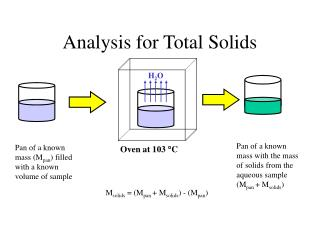DownloadDownload PresentationAnalysis for Total Solids

# Analysis for Total Solids

Download Presentation## Analysis for Total Solids

- - - - - - - - - - - - - - - - - - - - - - - - - - - E N D - - - - - - - - - - - - - - - - - - - - - - - - - - -
##### Presentation Transcript

1. H2O Pan of a known mass with the mass of solids from the aqueous sample (Mpan + Msolids) Pan of a known mass (Mpan) filled with a known volume of sample Oven at 103 C Analysis for Total Solids Msolids = (Mpan + Msolids) - (Mpan)

2. VS Furnace at 550 C Pan of a known mass with the mass of solids from the aqueous sample (Mpan + Msolids) Pan of a known mass with the mass of non-volatile solids from the aqueous sample (Mpan + MNVS) Analysis for Total Volatile and Non-Volatile Solids MNVS = (Mpan + MNVS) - (Mpan) MVS = (Mpan + MNVS) - (Mpan + MNVS)

3. TSS Separation of Dissolved and Suspended Solids Sample + DI rinse water Sample of a known volume containing all solids (Vsample) Filter of a known mass (Mfilter) TDS + H2O from sample + DI rinse water Known volume(s) of DI rinse water (VDI) Filtration Apparatus

4. H2O Filter of a known mass with mass of suspended solids (Mfilter + MSS) Filter of a known mass (Mfilter) with mass of suspended solids (MSS)and water (MH20) TSS TSS TSS TSS TSS Oven at 103 C NVSS VSS Filter of a known mass with mass of non-volatile suspended solids (Mfilter + MNVSS) Filter of a known mass with mass of suspended solids (Mfilter + MSS) Furnace at 550 C Analysis for SuspendedVolatile and Non-Volatile Solids (MSS) = (Mfilter + MSS) - (Mfilter) (MNVSS) = (Mfilter + MNVSS) - (Mfilter) (MVSS) = (Mfilter + MSS) - (Mfilter + MNVSS)

5. H2O * IMPORTANT * You must account for the dilution of the sample with DI water when calculating any of the dissolved solids concentrations. Analysis for Total Dissolved Solids Pan of a known mass filled with a known volume of sample from the filtration apparatus flask (Mpan + MTDS + H2O from sample + DI rinse water) Pan of a known mass with the mass of all dissolved solids from the aqueous filtrate sample (Mpan + MTDS) Oven at 103 C + Oven at 180 C (MTDS) = (Mpan + MTDS) - (Mpan)

6. * IMPORTANT * You must account for the dilution of the sample with DI water when calculating any of the dissolved solids concentrations. Analysis for DissolvedVolatile and Non-Volatile Solids VDS Pan of a known mass with the mass of non-volatile dissolved solids from the aqueous filtrate sample (Mpan + MNVDS) Pan of a known mass with the mass of all dissolved solids from the aqueous filtrate sample (Mpan + MTDS) Furnace at 550 C (MNVDS) = (Mfilter + MNVDS) - (Mfilter) (MVDS) = (Mfilter + MTDS) - (Mfilter + MNVDS)